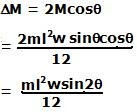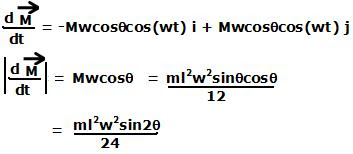Wednesday, March 11, 2009

Irodov Problem 1.282Consider an infinitesimally small piece of the rod at a distance x along the rod from point C of length dx. If the linear density (mass per unit length) of the rod is p then the mass of the piece will be pdx. The piece spins at a radius ofat a rate w and so has a linear velocity of. The momentum of the infinitesimally small piece of rod is given byand is directed perpendicular to the plane of axis and the rod directed into the plane.

Angular momentum w.r.t to C: The angular momentum of the piece with respect to C is then given by. The total angular momentum of the rod with respect to point C is given by,Angular momentum w.r.t to axis of rotation: The infinitesimally small piece is at a distancefrom the axis and so the angular momentum of this piece w.r.t to the axis of rotation is given by. The angular momentum of the entire rod w.r.t the axis of rotation is then given by,(b)The angular momentum vectors at two extremes of the rod's rotation are depicted in the figure beside. The net change in the angular momentum vector is given by,(c)While the magnitude of the angular momentum remains the same as the rod rotates, its direction keeps rotating as shown in the figure. Thus we have,The change in angular momentum vector is due to the torque provided on the axis given by,